[FPGA Tutorial] Seven-Segment LED Display on Basys 3 FPGA

This FPGA tutorial will guide you how to control the 4-digit seven-segment display on Basys 3 FPGA Board. A display controller will be designed in Verilog for displaying numbers on the 4-digit 7-segment LED display of the Basys 3 FPGA. Full Verilog code for the seven-segment LED display controller will also be provided.

The Basys 3 FPGA has a common-anode 4-digit 7-segment LED display as shown in the following figure.Seven anodes of the seven segments in a single LED are connected together to one common anode node while its cathodes are separate as shown in the following figure. DP-segment is to illuminate the dot so we omit the DP-segment for now since it is not contributing to the number value of the seven-segment display.To illuminate LED segments such as A-G, anodes of these segments need to be at the 'high' logic level and cathodes should be at the 'low' logic level. Thus, the common anode node should be high to activate a single seven-segment LED display. The logic levels of cathodes can be varying from low (illuminated) to high(un-illuminated) to show different decimal values on a single 7-segment LED display as follows.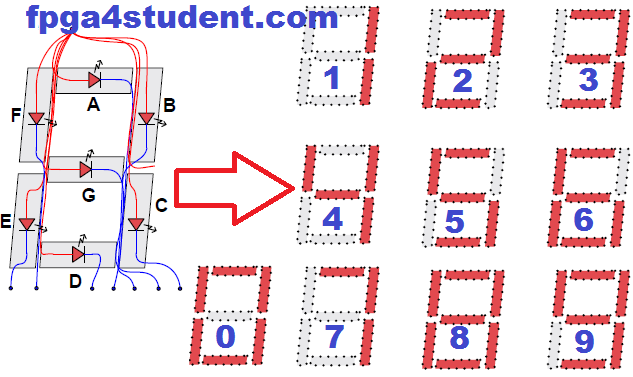As shown in the figure above, we can display the numbers from zero to nine on the seven-segment LED display by turning on and off the seven segments of the single seven-segment LED display. For example, to show number "1" on the LED, the segments A, F, G, E, and D are un-illuminated or their cathodes should be high, and B-C segments are illuminated or their cathodes should be low. Similarly, we can obtain the following decoder table for displaying ten numbers on seven-segment LED display:For now, we know how to display numbers on a single seven-segment LED display on Basys 3 FPGA board. However, the cathodes of four seven-segment LEDs on Basys 3 are not separate but connected together as shown in the following figure.Thus, to display 4 different numbers on the 4-digit seven-segment LED display, we have to control the cathodes (CA-CG) of the four seven-segment LEDs separately by activating the four seven-segment LEDs at different times. For example, when we activate LED 1 by driving A1 high and the other three LEDs (LED 2, LED 3, LED 4) are deactivated (A2, A3, and A4 not driven), the cathode pattern (CA-CG) will be used for displaying numbers on LED 1. Similarly, LED 2 - LED 4 can be displayed by using the same way at different times. As shown in the figure above, we can control activating four seven-segment LEDs by using 4 PNP transistors AN0-AN3. When a transistor is on or the Base terminal (W4, U4, V4, or U2) is low, the corresponding anode is driven high to activate or enable the corresponding seven-segment LED.

In this FPGA tutorial, a seven-segment LED display controller is designed for displaying numbers on the four-digit 7-segment LED display of the Basys 3 FPGA board. The following is the timing diagram for the seven-segment LED display controller on Basys 3 FPGA: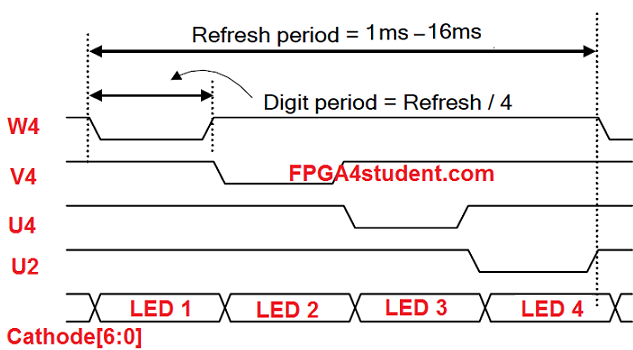Below is an example timing diagram for displaying "1234" on the 4-digit 7-segment LED display of Basys 3 FPGA board with the refresh rate of 60Hz or period of 16ms: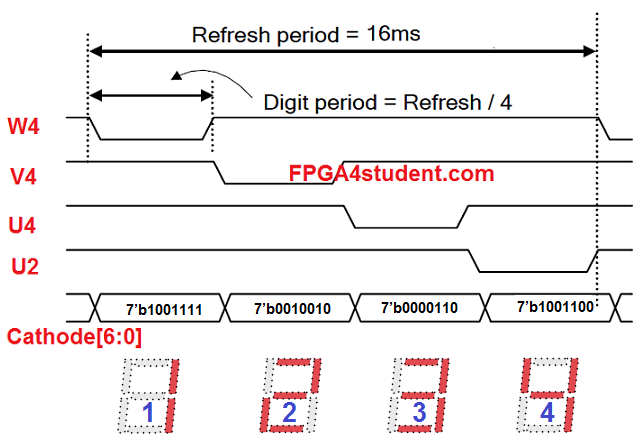Now, we know how to control and display numbers on the four-digit seven-segment LED display on Basys 3 FPGA. Next, let's design the 7-segment LED display controller.

The Basys 3 FPGA has a clock source of 100MHz and we need a 1ms-16ms refresh period or a 1KHz-60Hz refresh rate. I will choose a refresh period of 10.5ms (digit period = 2.6ms) so that we can use a 20-bit counter for creating the refresh period with the first 2 MSB bits of the counter for creating LED-activating signals (digit period of 2.6ms) as shown in the timing diagram above.

Below is an example Verilog code for creating the refresh signal and LED-activating signals:

`reg [19:0] refresh_counter; `
```// the first 18-bit for creating 2.6ms digit period
// the other 2-bit for creating 4 LED-activating signals```
`wire [1:0] LED_activating_counter; `
`// count        0    ->  1  ->  2  ->  3`
```// activates    LED1    LED2   LED3   LED4
// and repeat
always @(posedge clock_100Mhz or posedge reset)
begin
if(reset==1)
refresh_counter <= 0;
else
refresh_counter <= refresh_counter + 1;
end
assign LED_activating_counter = refresh_counter[19:18];
```
After that, we need to generate the anode signals (W4, V4, U4, U2) for the four-digit 7-segment LED display based on the LED-activating counter. The LED-activating counter will repeatedly count from zero to three for continuously activating and updating the four seven-segment LEDs.

Below is an example Verilog code for creating the anode signals and updating values of the four 7-segment LEDs on Basys 3 FPGA:

```    // anode activating signals for 4 LEDs
// decoder to generate anode signals
always @(*)
begin
case(LED_activating_counter)
2'b00: begin
Anode_Activate = 4'b0111;
// activate LED1 and Deactivate LED2, LED3, LED4
LED_BCD = displayed_number[15:11];
// the first hex-digit of the 16-bit number
end
2'b01: begin
Anode_Activate = 4'b1011;
// activate LED2 and Deactivate LED1, LED3, LED4
LED_BCD = displayed_number[10:8];
// the second hex-digit of the 16-bit number
end
2'b10: begin
Anode_Activate = 4'b1101;
// activate LED3 and Deactivate LED2, LED1, LED4
LED_BCD = displayed_number[7:4];
// the third hex-digit of the 16-bit number
end
2'b11: begin
Anode_Activate = 4'b1110;
// activate LED4 and Deactivate LED2, LED3, LED1
LED_BCD = displayed_number[3:0];
// the fourth hex-digit of the 16-bit number
end
default:begin
Anode_Activate = 4'b0111;
// activate LED1 and Deactivate LED2, LED3, LED4
LED_BCD = displayed_number[15:11];
// the first hex-digit of the 16-bit number
end
endcase
end
```

Last but not least, the example Verilog code for BCD to 7-segment decoder based on the decoder table above:

```reg[6:0] LED_out;
// Cathode patterns of the 7-segment LED display
always @(*)
begin
case(LED_BCD)
4'b0000: LED_out = 7'b0000001; // "0"
4'b0001: LED_out = 7'b1001111; // "1"
4'b0010: LED_out = 7'b0010010; // "2"
4'b0011: LED_out = 7'b0000110; // "3"
4'b0100: LED_out = 7'b1001100; // "4"
4'b0101: LED_out = 7'b0100100; // "5"
4'b0110: LED_out = 7'b0100000; // "6"
4'b0111: LED_out = 7'b0001111; // "7"
4'b1000: LED_out = 7'b0000000; // "8"
4'b1001: LED_out = 7'b0000100; // "9"
default: LED_out = 7'b0000001; // "0"
endcase
end
```
The design for 7-segment LED display controller is basically completed. Let's use the controller to display a 16-bit counting number on the four-digit seven-segment LED display of the Basys 3 FPGA board with the counting period of 1 second.

Full Verilog code of the seven-segment LED display controller for displaying counting numbers on Basys 3 FPGA:

```// fpga4student.com: FPGA projects, Verilog projects, VHDL projects
// FPGA tutorial: seven-segment LED display controller on Basys  3 FPGA
module Seven_segment_LED_Display_Controller(
input clock_100Mhz, // 100 Mhz clock source on Basys 3 FPGA
input reset, // reset
output reg [3:0] Anode_Activate, // anode signals of the 7-segment LED display
output reg [6:0] LED_out// cathode patterns of the 7-segment LED display
);
reg [26:0] one_second_counter; // counter for generating 1 second clock enable
wire one_second_enable;// one second enable for counting numbers
reg [15:0] displayed_number; // counting number to be displayed
reg [3:0] LED_BCD;
reg [19:0] refresh_counter; // 20-bit for creating 10.5ms refresh period or 380Hz refresh rate
// the first 2 MSB bits for creating 4 LED-activating signals with 2.6ms digit period
wire [1:0] LED_activating_counter; ```
```                 // count     0    ->  1  ->  2  ->  3
// activates    LED1    LED2   LED3   LED4
// and repeat
always @(posedge clock_100Mhz or posedge reset)
begin
if(reset==1)
one_second_counter <= 0;
else begin
if(one_second_counter>=99999999)
one_second_counter <= 0;
else
one_second_counter <= one_second_counter + 1;
end
end
assign one_second_enable = (one_second_counter==99999999)?1:0;
always @(posedge clock_100Mhz or posedge reset)
begin
if(reset==1)
displayed_number <= 0;
else if(one_second_enable==1)
displayed_number <= displayed_number + 1;
end
always @(posedge clock_100Mhz or posedge reset)
begin
if(reset==1)
refresh_counter <= 0;
else
refresh_counter <= refresh_counter + 1;
end
assign LED_activating_counter = refresh_counter[19:18];
// anode activating signals for 4 LEDs, digit period of 2.6ms
// decoder to generate anode signals
always @(*)
begin
case(LED_activating_counter)
2'b00: begin
Anode_Activate = 4'b0111;
// activate LED1 and Deactivate LED2, LED3, LED4
LED_BCD = displayed_number/1000;
// the first digit of the 16-bit number
end
2'b01: begin
Anode_Activate = 4'b1011;
// activate LED2 and Deactivate LED1, LED3, LED4
LED_BCD = (displayed_number % 1000)/100;
// the second digit of the 16-bit number
end
2'b10: begin
Anode_Activate = 4'b1101;
// activate LED3 and Deactivate LED2, LED1, LED4
LED_BCD = ((displayed_number % 1000)%100)/10;
// the third digit of the 16-bit number
end
2'b11: begin
Anode_Activate = 4'b1110;
// activate LED4 and Deactivate LED2, LED3, LED1
LED_BCD = ((displayed_number % 1000)%100)%10;
// the fourth digit of the 16-bit number
end
endcase
end
// Cathode patterns of the 7-segment LED display
always @(*)
begin
case(LED_BCD)
4'b0000: LED_out = 7'b0000001; // "0"
4'b0001: LED_out = 7'b1001111; // "1"
4'b0010: LED_out = 7'b0010010; // "2"
4'b0011: LED_out = 7'b0000110; // "3"
4'b0100: LED_out = 7'b1001100; // "4"
4'b0101: LED_out = 7'b0100100; // "5"
4'b0110: LED_out = 7'b0100000; // "6"
4'b0111: LED_out = 7'b0001111; // "7"
4'b1000: LED_out = 7'b0000000; // "8"
4'b1001: LED_out = 7'b0000100; // "9"
default: LED_out = 7'b0000001; // "0"
endcase
end
endmodule```
Pin constraint file for the four-digit seven-segment LED display on Basys 3 FPGA:
```# Clock signal
set_property PACKAGE_PIN W5 [get_ports clock_100Mhz]
set_property IOSTANDARD LVCMOS33 [get_ports clock_100Mhz]
set_property PACKAGE_PIN R2 [get_ports reset]
set_property IOSTANDARD LVCMOS33 [get_ports reset]
#seven-segment LED display
set_property PACKAGE_PIN W7 [get_ports {LED_out}]
set_property IOSTANDARD LVCMOS33 [get_ports {LED_out}]
set_property PACKAGE_PIN W6 [get_ports {LED_out}]
set_property IOSTANDARD LVCMOS33 [get_ports {LED_out}]
set_property PACKAGE_PIN U8 [get_ports {LED_out}]
set_property IOSTANDARD LVCMOS33 [get_ports {LED_out}]
set_property PACKAGE_PIN V8 [get_ports {LED_out}]
set_property IOSTANDARD LVCMOS33 [get_ports {LED_out}]
set_property PACKAGE_PIN U5 [get_ports {LED_out}]
set_property IOSTANDARD LVCMOS33 [get_ports {LED_out}]
set_property PACKAGE_PIN V5 [get_ports {LED_out}]
set_property IOSTANDARD LVCMOS33 [get_ports {LED_out}]
set_property PACKAGE_PIN U7 [get_ports {LED_out}]
set_property IOSTANDARD LVCMOS33 [get_ports {LED_out}]```
```set_property PACKAGE_PIN U2 [get_ports {Anode_Activate}]
set_property IOSTANDARD LVCMOS33 [get_ports {Anode_Activate}]
set_property PACKAGE_PIN U4 [get_ports {Anode_Activate}]
set_property IOSTANDARD LVCMOS33 [get_ports {Anode_Activate}]
set_property PACKAGE_PIN V4 [get_ports {Anode_Activate}]
set_property IOSTANDARD LVCMOS33 [get_ports {Anode_Activate}]
set_property PACKAGE_PIN W4 [get_ports {Anode_Activate}]
set_property IOSTANDARD LVCMOS33 [get_ports {Anode_Activate}]```
Now, create a new project in Vivado, choose the device part number of XC7A35T-1CPG236C for Artix-7 FPGA on Basys 3 FPGA board. Then, add the source and constraint files, and generate the bitstream. Program the FPGA using the bit stream and see how it works on the Basys 3 FPGA board.

Demo video for seven-segment LED display controller on Basys 3 FPGA board:

Here is the full VHDL code for seven-segment display on Basys 3 FPGA.
Recommended FPGA projects for students:

1.Hi;
I didn't understand how 19-bits = 190Hz?

1.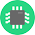19 bit refresh counter can count up to 2^19 - 1 = 524287. The clock input is 100Mhz. So you can obtain the refresh rate = 100,000,000/524,287 = 190 Hz.

2.Ahaaa, but if I do same to the one second counter the result is not pure 1 sec !
100MHz / 2^27 = 0.75 ---> 1/0.75 = 1.33 sec
Is that calc Ok? and you cant get pure 1 sec ? but how if I want a precise clock in micro sec or even nano sec?
Thanks

3.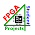If you use a 27-bit counter, it will count up to 134,217,727. Therefore, you need to reset the counter to 0 when it reaches 100,000,000. Then, you will get 1 second clock.

4.So, you are generating a 190Hz refresh rate by benefiting the overflow of the variable when it counts up to 524287 + 1 ?

5.The Basys 3 FPGA has a clock source of 100MHz and we need a 1ms-16ms refresh period or a 1KHz-60Hz refresh rate. I will choose a refresh period of 10.5ms (digit period = 2.6ms) so that we can use a 20-bit counter for creating the refresh period with the first 2 MSB bits of the counter for creating LED-activating signals (digit period of 2.6ms) as shown in the timing diagram above.

2.how to counnt up to 99 and go back to 0

1.Make a mod 100 counter using 8 bit counter and when the counter reaches 100 (01100100) clear it to zero by taking the Q(6),Q(5) and Q(2) bit of output to the input of and3 gate and feeding that output to clear of counter ..

3.sir
please provide tutorial on interfacing of lcd(digilent pmodclp) with basys 3 fpga.

4.This comment has been removed by the author.

1.For example: You have a 4-digit number: 1234, that code will take out the number "4" for displaying.

5.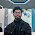Hello, Where can i get the full circuit diagram of this?

1.use Vivado to generate the full RTL schematic

6.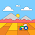Hi, i have 16 bit output, i want do display the value to 4 seven segment in hex.Do have any idea to solve this problem

1.The provided code is to display 16-bit number on the 4-digit 7-segment LED display. Exactly what you need.